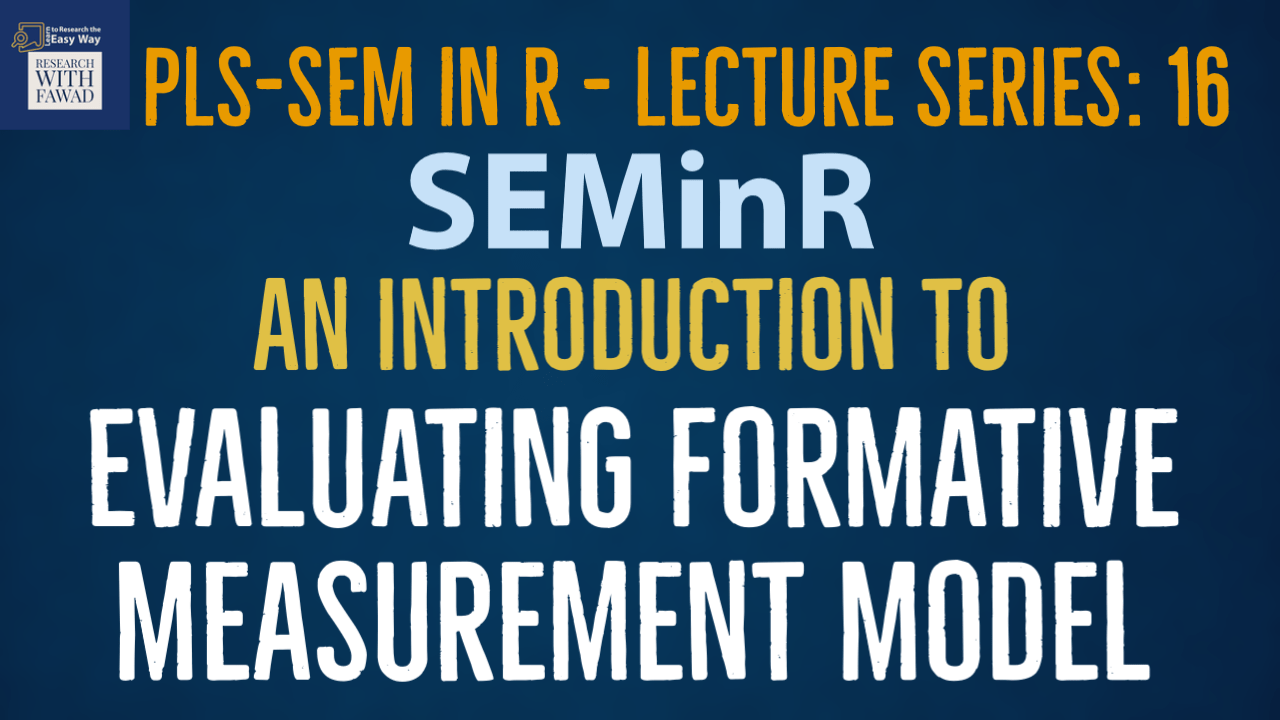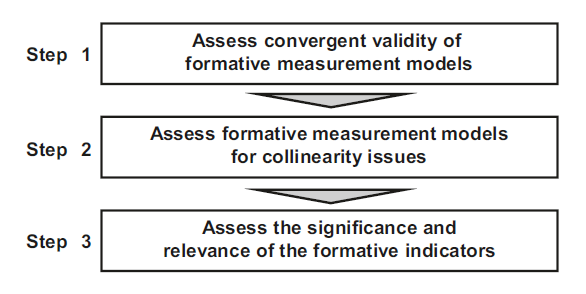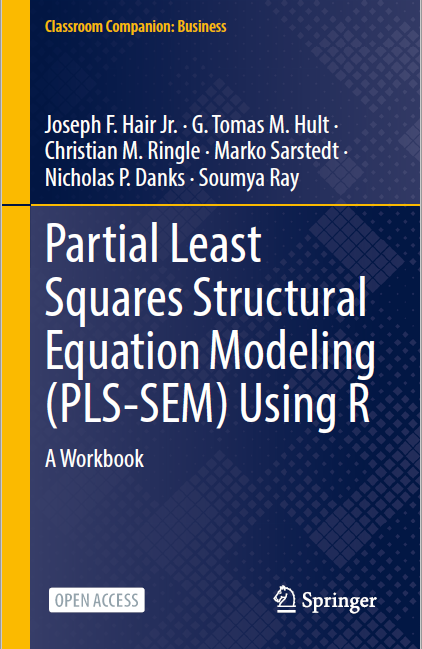# An Introduction to Evaluating Formative Measurement Model.### SEMinR Lecture Series

This session is focused on introducing how to evaluate a formative measurement model in SEMinR.

## Evaluating Formative Measurement Model

• PLS-SEM is the preferred approach when formatively specified constructs are included in the PLS path model (Hair, Risher, Sarstedt, & Ringle, 2019).
• In this part of the series, I discuss the key steps for evaluating formative measurement models (Fig.). Relevant criteria include the assessment of Convergent validity,indicator collinearity, and Statistical significance and relevance of the indicator weights.
• Next, I will introduce key criteria and their thresholds and illustrate their use with an example.## The Example

• The proposed model has three constructs (Vision, Development, and Reward) measured formatively that impact a reflectively measured construct (Collaborative Culture).
• The three constructs are formative constructs, estimated with mode_B, while Collaborative Culture is reflective construct, estimated with mode_A.
• The weights parameter of the composite() function is set by default to mode_A. Thus, when no weights are specified, the construct is estimated as being reflective.
• Alternatively, we can explicitly specify the mode_A setting for reflectively measured constructs or the mode_B setting for formatively measured constructs.
• Once the model is set up, we use the estimate_pls() function to estimate the model, this time specifying the measurement_model and structural_model.
• Finally, we apply the summary() function to the estimated SEMinR model object simple_model and store the output in the summary_simple object.

## The Code

``````library(seminr)
# Create measurement model
simple_mm <- constructs(
composite("Vision", multi_items("VIS", 1:4), weights = mode_B),
composite("Development", multi_items("DEV", 1:7), weights = mode_B),
composite("Rewards", multi_items("RW",1:4), weights = mode_B),
composite("Collaborative Culture", multi_items("CC", 1:6)))
# Create structural model
simple_sm <- relationships(
paths(from = c("Vision","Development","Rewards"), to = "Collaborative Culture"))
# Estimate the model
simple_model <- estimate_pls(data = datas,
measurement_model = simple_mm,
structural_model = simple_sm,
missing = mean_replacement,
missing_value = "-99")
# Summarize the model results
summary_simple <- summary(simple_model)
``````

## Results

• It is always a good idea to assess the missing values. The number of missing observations is reported in the descriptive statistic object nested within the summary return object. This report can be accessed by inspecting the summary as
``summary_simple\$descriptives\$statistics``
• When the PLS-SEM algorithm stops running, check whether the algorithm converged (Hair et al., 2022, Chap. 3). For this example, the PLS-SEM algorithm will stop when the maximum number of 300 iterations or the stop criterion of 1.0E-7 (i.e., 0.0000001) is reached. To do so, it is necessary to inspect the simple_model object summary output by using the \$ operator:
``````# Iterations to converge
summary_simple\$iterations``````
• The results show that the model estimation converged after eight iterations.

## Reflective Measurement Model Assessment

• An important characteristic of PLS-SEM is that the model estimates will change when any of the model relationships or variables are changed.
• We thus need to reassess the reflective measurement models to ensure that this portion of the model remains valid and reliable before continuing to evaluate the four new exogenous formative constructs.
• We then follow the reflective measurement model assessment procedure.

## Reference

Hair Jr, J. F., Hult, G. T. M., Ringle, C. M., Sarstedt, M., Danks, N. P., & Ray, S. (2021). Partial Least Squares Structural Equation Modeling (PLS-SEM) Using R: A Workbook.The tutorials on SEMinR are based on the mentioned book. The book is open source and available for download under this link.